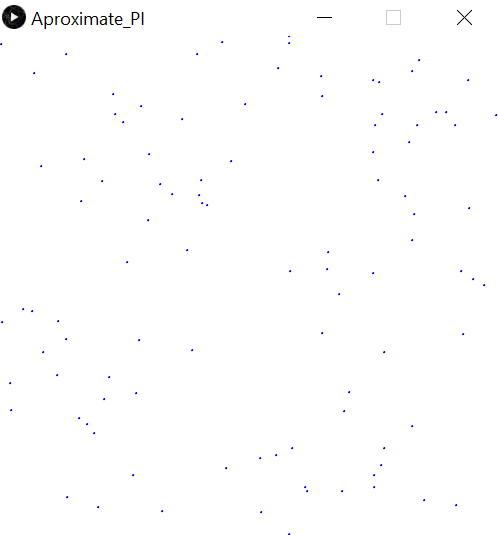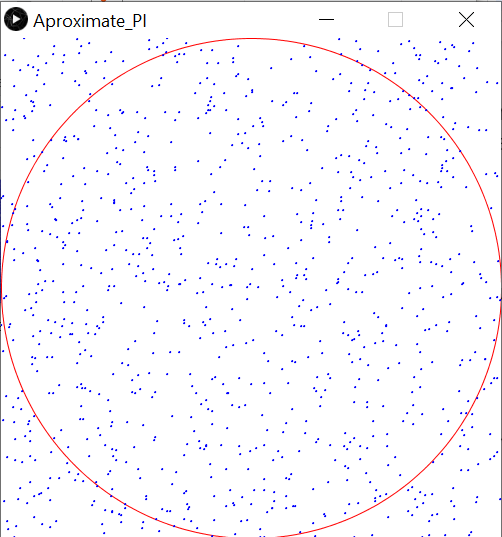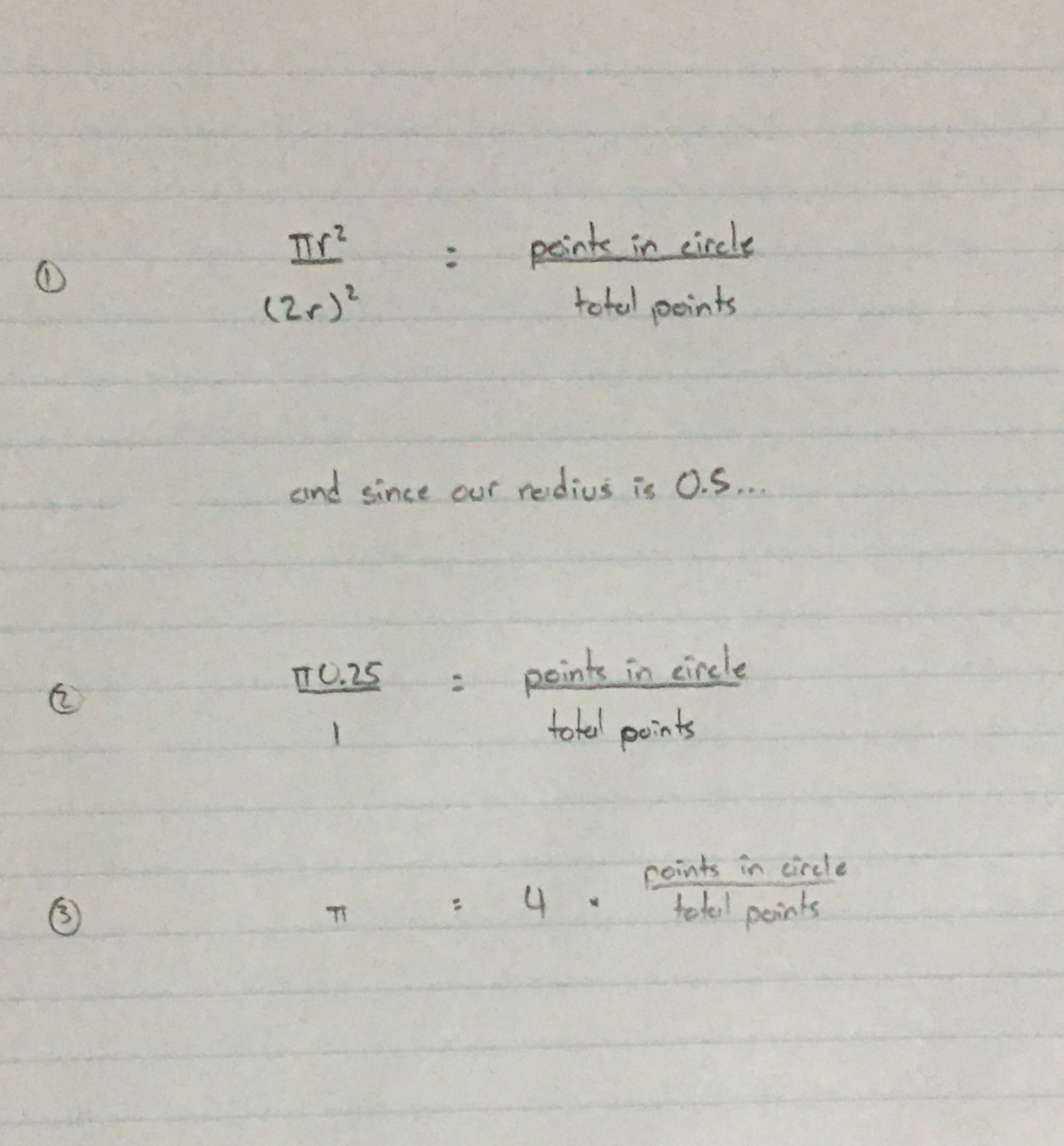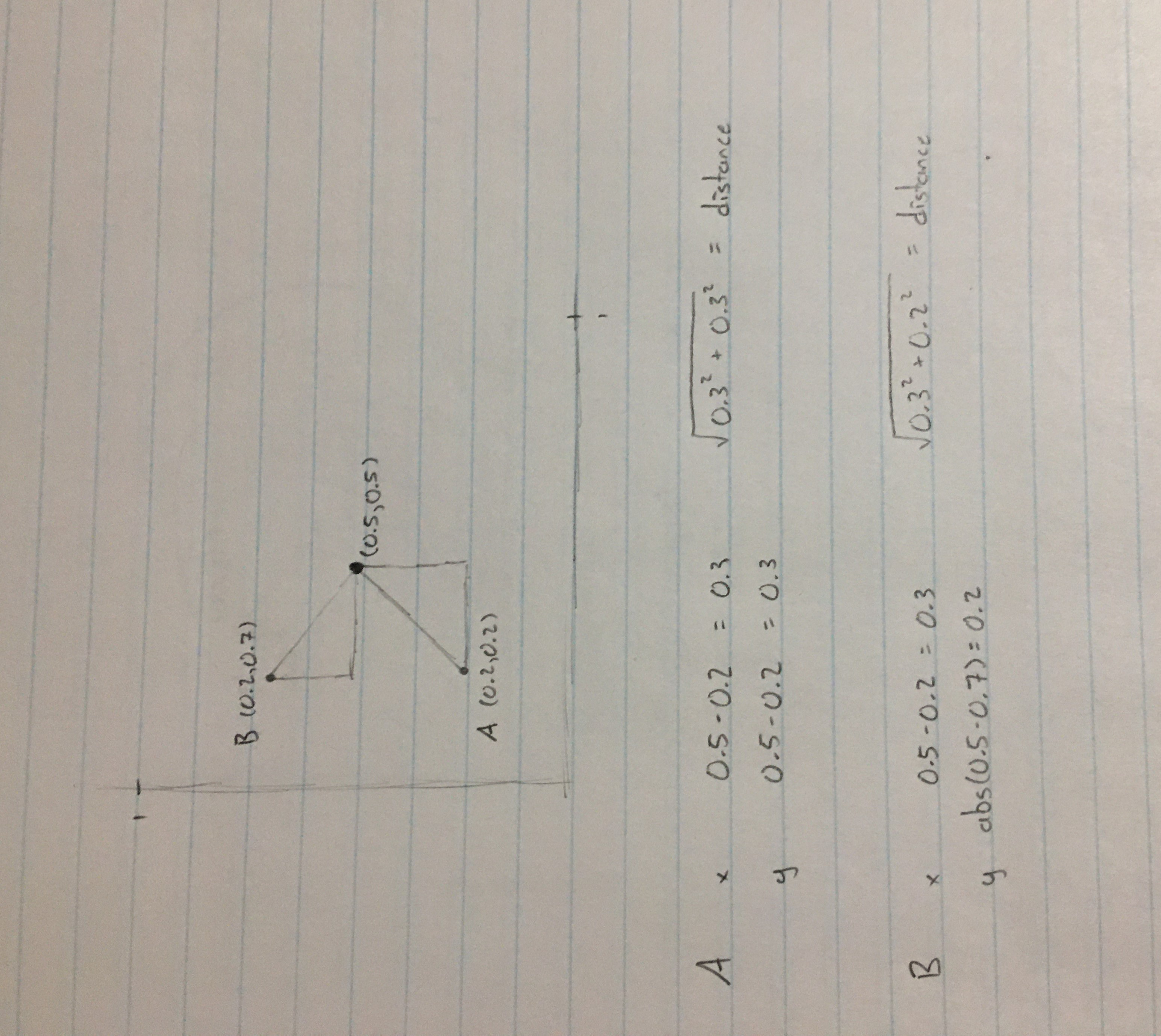The Monte Carlo method explained

Thanks to this youtuber for first introducing me to this problem.

In this tutorial, we’ll be solving a problem. Now this is actually one of my favourite problems cause it’s so ridiculous it makes absolutely no sense. Here’s the problem:

"Giving a bunch of randomly generated numbers between the values of 0 and 1...

approximate PI"

I know, this sounds absolutely nuts! Like what? Calculate PI using a bunch of numbers? How?? That's what I first said when I saw this problem. Well, let’s solve it!

Well first, let’s think about this problem. So you have an array of randomly generated values, and you’re trying to calculate PI. So let’s think, what do we use PI for? Or think about where you would see PI in the math world.

Maybe the area of a circle?

Hmm… we’re getting somewhere. But how are we going to relate a circle to a bunch of numbers? Well, what thing can have shapes and numbers?

Coordinate grid?

Try to take two numbers, and turn them into coordinates (first number is the x value, second is the y). Then plot them on a grid, and you should have something that looks like this:Oh, and the circle:Okay, but how do we calculate PI? Well, what formulas can we make of this? There isn’t a formula that solves for PI right away like: `PI = something`, however, there are formulas which contain PI, and then we can just solve for PI! So what formulas contain PI? Maybe:

``(𝜋r^2) / (2𝜋)^2 = (number of points in the circle) / (number of total points)``

So we did this by taking the area of the circle, and dividing that by the total number of points (think of the grid as a square). Remember, the points in shapes represent the areas of that shape. Well, like, if there’s 10 points in the circle, it doesn’t mean the circle’s area is 10, it is just a ratio of the area. And the more points we have, the better we can then represent the area, and the more accurate answer we have!

But wait, we must first solve the equation for PI (sorry if it’s a little blurry).And there we go! We have PI is equal to points inside the circle divided by the total points, and multiplied by 4!

But how do we know if a point is in the circle? Well we can get the distance between that point and the center of the circle. But how do we find the distance between a point (x, y) and the center of the circle (0.5, 0.5)? Well we have to use the Pythagorean theorem!So what I did was create two points, A and B, and basically just did some math. Oh, and remember to take the absolute value of the distance since we don’t want negatives!

So let’s code this (C++)!

``````#include <iostream>
#include <cmath>
#include <ctime>

int main()
{
srand(time(NULL)); // used to generate different random numbers

double total = 100; // total number of points
double inside = 0; // number of points in the circle

double x, y; // coordinates

for (int a = 0; a < total; ++a)
{
// generates a random number between 0 and 100, and divides
// it by 100 to make a decimal between 0 and 1

x = (rand() % 100) / 100.0;
y = (rand() % 100) / 100.0;

// using the Pythagorean theorem to calculate the distance
// between the center of the circle and the point

x = abs(0.5 - x);
y = abs(0.5 - y);

if (sqrt(x*x + y*y) <= 0.5) // 0.5 is the radius of the circle
inside += 1;
}

std::cout << 4 * (inside / total); // displaying the result
}``````

Output:

``3.2``

Close. But can we do better? Instead of creating 100 points, what about 1000?

Output:

``3.116``

Better. But how close can we get?

``````10’000      - 3.1236
100’000     - 3.13216
1’000’000   - 3.13904``````

Close enough. It really depends on your compiler on how big you can get.

Oh, and as a bonus, I did this in Processing and made some visuals! Cause who doesn’t like visuals?

``````long total = 1000; // total number of points that will be created
float inside = 0; // number of points inside the circle

int p1; // point 1 - x coordinate of dot
int p2; // point 2 - y coordinate of dot

void setup()
{
/* don't worry about any of the below code */

size(500, 500); // creates screen
background(255); // sets the colour of the background to white

noFill(); // makes circle transparent so we can see points inside
stroke(255, 0, 0); // sets circle outline to red
ellipse(250, 250, 500, 500); // draws circle

stroke(0, 0, 255); // sets the colour of the dots to blue

/* don't worry about any of the above code */

for (int a = 0; a < total; ++a) // this loop creates dots
{
p1 = int(random(0, 500)); // random x coordinate
p2 = int(random(0, 500)); // random y coordinate

ellipse(p1, p2, 1, 1); // draws dot at random x and y coordinates

// if the distance between the dot and the center is below 500 (radius of the circle)
if (dist(p1, p2, 250, 250) <= 250)
inside += 1;
}

print(4 * (inside / total)); // displays result to the console
}``````

Oh, and I used a 500 by 500 grid instead of a 1 by 1 since it’s easier to visualize. But the same idea. Also, there’s this thing called the `dist()` function in Processing that calculates the distance for you! Why can’t C++ have cool things like that? :(

Output:

``````(open the links if you want to see the pictures)

1000 - 3.148

10’000 - 3.1356

100’000 - 3.14716
(you can barely see the circle!)

I also modified this a little bit and created a loop, and after a few minutes of calculating, the closest I could come was `3.14156`. The actual value of PI is `3.14159`! So close!

Well, I’ll call that a success! We approximated PI using just random numbers!

Now what we did here was called the Monte Carlo method. We used a bunch of random samples to calculate a numeric value. Now in this tutorial, I used the Monte Carlo method to calculate PI, but you can you it for all sorts of things, even estimating stock prices!

Maybe I'll post another tutorial later explaining another cool thing we can do with this.

Well, hope you guys learned something cool today, and don’t forget to like!

You are viewing a single comment. View All
DynamicSquid (4551)

@StudentFires Isn't that why I have to use a time seed? `srand(time(0))`